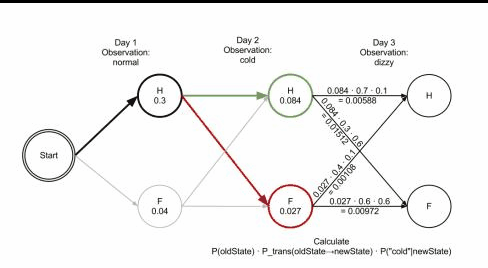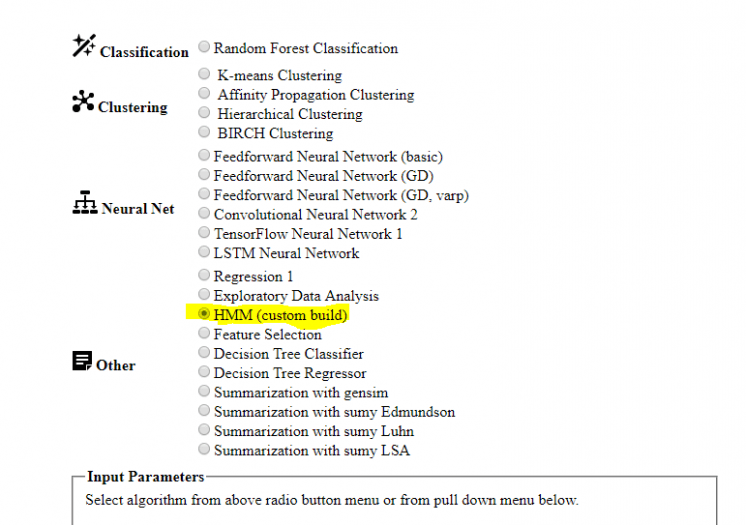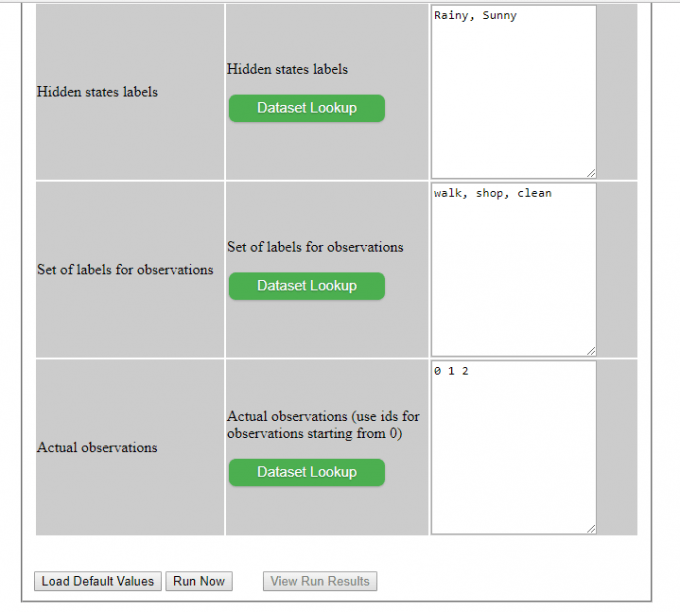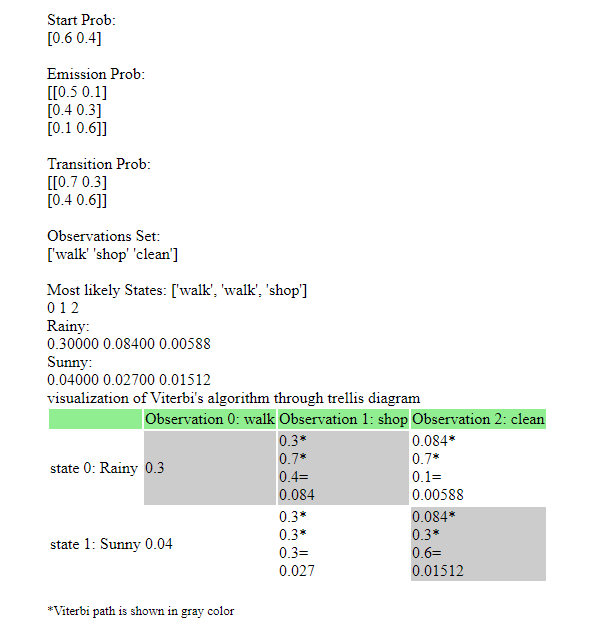## Hidden Markov Models and Trellis Diagram

The Viterbi path is the most likely sequence of hidden states that produce a sequence of observed events. We can calculate Viterbi path using Viterbi algorithm. The focus of this post will be how to visualize Viterbi path for HMM (Hidden Markov Models) using trellis diagram. Finding Viterbi path provides the answer to decoding question in HMM – given observations and states transitions find most likely states.

In many papers and texts we can find trellis diagrams are utilized to solve or visualize the above decoding problem. Trellis structures can be represented with the help of table that have number of rows equal to number of states and number of columns equal to number of observations.

When we move from one state in one column to another state in adjacent column we use transition probability matrix.

The likelihood of the state sequence given the observation sequence can be found by simply following the path in the trellis diagram, multiplying the observation and transition likelihoods.

Below are decoding examples of calculating probabilities in Viterbi algorithm.Trellis Diagram Step2Trellis Diagram Step3Visualization of Viterbi’s path through trellis diagram

Source: Wikipedia An example of HMM

Animated examples like above looks good but what if you want to plug your data and see results?

## Calculating Viterbi Path Online

Here is how you can calculate Viterbi algorithm and visualize Viterbi path using the online tool from this blog site. The site is using code from tensorflow_hmm provided by Zach Dwiel.  The code actually has two implementations – one is using numpy array and another is using tensorflow library.

I added visualization Viterbi path through trellis table. With this addition we can understand more and easy how the path was calculated. And also we can track the path through different states and observations. Below is the sample of output for the table with calculated Viterbi path. The Viterbi path is represented by gray cells, states: 0,0,1Viterbi Path Visualization

Below are the steps how to use Online Machine Learning Algorithms tool:

1. Access Online Machine Learning Algorithms and select HMM as shown on below screenshot. HMM highlighted in yellow.Online Machine Learning Algorithms Tool Step1

2. Input the data that you want to run. Or click Load Default Values to run with preconfigured test example. Use this button to see input data format.Online Machine Learning Algorithms Tool Step1

3. Click Run now.
4. Click View Run Results.

5. Click Refresh Page button on this new page , you maybe will need click few times untill data output show up. Usually it takes less than 1 min, but it will depend how much data you need to process.
Scroll to the bottom page to see calculations.Online Machine Learning Algorithms Tool Step3

Hope you will find useful online tool for Visualization of Viterbi Path for Hidden Markov Models and will give try to run HMM for your data or default data. Feel free to post in the comments box questions or suggestions.

References
1. An example of HMM
2. tensorflow_hmm
3. Online Machine Learning Algorithms Tool